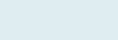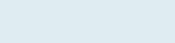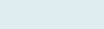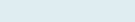# Find Age from Current Date

After reading this article, you will able to calculate the age between two dates i.e. today’s date or certain specific date and birth date with the exact number of years, months and days passed in the same cell.

Query: We have a birth date and today’s date. Our task is to get age based on two dates (given) after rounding.

Formula to implement:

=ROUND(YEARFRAC(C2, TODAY(), 1), 0)

Functions applicable:

Round: The Excel ROUND function returns a number after rounding a supplied number or time to a specific number of digits.

Yearfrac: The Excel YEARFRAC function calculates the fraction of years that have elapsed within a given range of dates.

Today: The Excel TODAY function returns the current date in the selected cell.

Formula Explanation (step by step)

Excel starts calculating innermost parenthesis than work outward. You can also debug the formula using a shortcut F9 function key under the formulas tab.

Let’s break the formula into multiple small steps to understand its application.

First formula:

Syntax: =TODAY()

Step1:Result: 43880

It will give present date (19-02-2020) in serial number form.

Second formula:

YEARFRAC(C2, TODAY(), 1)

Syntax: YEARFRAC (start_date, end_date, [basis])

Step 2:Result:From step 1 we already get the current date, now it returns the number of years on the basis of both dates.

Putting steps 1,2 in the third formula.

Third formula:

=ROUND(YEARFRAC(C2, TODAY(), 1), 0)

Syntax: =ROUND (number, num_digits)

Step 3:Result: 24

## Age in years, months and days

Query: If the user wishes to get age in separate form like total years, months and days spend based on current date.

Formula to implement:

=DATEDIF(C2,C3,”Y”)&” Years, “&DATEDIF(C2,C3,”YM”)&” Months, “&DATEDIF(C2,C3,”MD”)&” Days”

Function applicable:

DATEDIF: The Excel DATEDIF is an unknown compatibility function that can be used to calculate the difference between two dates.

Formula explanation (step by step)

=DATEDIF(C2,C3,”Y”)&” Years, “&DATEDIF(C2,C3,”YM”)&” Months, “&DATEDIF(C2,C3,”MD”)&” Days”

Syntax: =DATEDIF(start_date,end_date,unit)

Step 1: DATEDIF(C2,C3,”Y”)&” Years

Result:24 Years

We will get total number of years (24) along with text “Years”.

Step 2: DATEDIF(C2,C3,”YM”)&” Months

Result: 2 months

Now we will get the total number of months (2) with text “Months”.

Step 3: DATEDIF(C2,C3,”MD”)&” Days”

Result: 4 days

After getting years and months with text then it returns the count of the number of days spend from the current date.

Joining all three steps together in step 4 to get answers total time passed in the same cell.

Step 4: =DATEDIF(C2,C3,”Y”)&” Years, “&DATEDIF(C2,C3,”YM”)&” Months, “&DATEDIF(C2,C3,”MD”)&” Days”

Result: 24 years, 2 months, 4 days.

It will give total time passed in years, months and days in the same cell with text.

Please review once how Print is done simplest way in Excel.

Note: “&” operator in Excel is a type of concatenate which is used to join two strings, values or results.